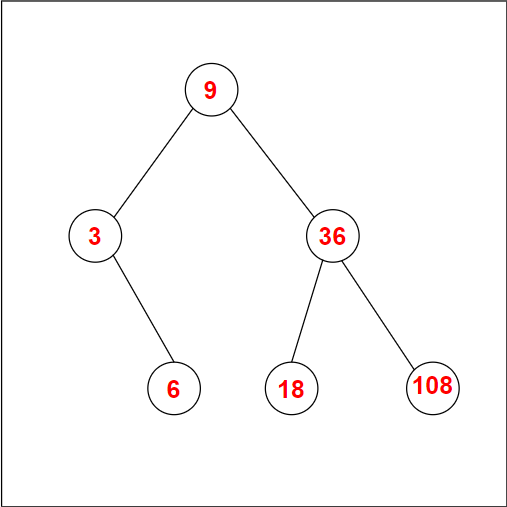# Check for Binary Search Tree

## Question:

Given an array, A of size N. Find whether it is possible to make a Binary Search Tree with elements of A such that the greatest common divisor of any two vertices connected by a common edge is > 1. Print Yes if possible, otherwise print No.

Examples:

Alert Ninjas:  Don’t stop now! Enroll in the Competitive Programming course today and start coding. Learn the art of writing time and space-efficient code and compete in code competitions worldwide.

1.

Explanation: One of the possible BTS is given below. Here vertices of every edge have GCD > 1.2.

Explanation: GCD of 2 and 47 is 1.

Recommended: Please try to solve it yourself before moving on to the solution.

## Solution:

### Approach1:

The idea is to use Dynamic Programming (DP).

Let DP(l, r, root) be a DP array with values 0 or 1 determining if it is possible to create a BST root at the root from the sub-segment [ l, r ] of the given array.

Calculating  DP(l, r, root) requires extracting the (leftRoot) root of the left subtree from [l..root – 1] and (rightRoot) root of the right subtree from [root + 1..right] in the following manner:

• gcd(aroot, arightRoot) > 1
• gcd(aroot, aleftRoot) > 1
• DP(l, root-1, leftRoot) = 1
• DP(root+1, r, rightRoot) = 1

In DP(l, r, root), l can take n values, r can take n values, and root can also take n values. Therefore there are n3 DP states.

Also, the transition time for DP will be O(n). Hence, a total of n4 time complexity of this solution.

### Better Approach

Let’s define DP differently; let DPNew(l, r, state) be a DP, where a state can be 0 or 1.

Here we can calculate DP(l, r, root) from DPNew(l, root - 1, 1) and DPNew(root + 1, r, 0).

Here l can take n values, r can take n values, and state can take two values. Therefore there are n2 DP states.

Also, the transition time for DP will be O(n). Hence, it has a total of n3 time complexity which is enough to pass.

C++

Output:Time Complexity: O(N3)

Q1: What is a Binary Search Tree in data structure?
Ans1:The Binary Search Tree data structure is a node-based binary tree with the following properties:

• The left subtree contains only nodes whose key value is smaller than the root's key value.
• The right subtree contains only nodes whose key value is greater than the root's key value.
• Each left and right subtree must be a Binary Search Tree.

Q2: What is a Binary Tree used for?
Ans2: Binary Trees are mainly used for searching and sorting since they allow hierarchical data storage. Some common operations on a Binary Tree include Insertion, deletion, and traversal.

Q3: Where can I practice Binary Search Tree Questions?
Ans3: Read and Practice important interview-related Binary Search Tree questions here. It is free and covers basic to advanced coding questions on Binary Search Tree.

## Key Takeaways

This article solved a DP-based problem where an array was given and a condition to satisfy; we needed to find if a Binary Search Tree can be created from the given array or not.  We solved the problem with a DP approach taking o(n3) time complexity.

You can practice more DP questions from here.

If you are new to DP and want to do a course covering all the DP concepts from basics to advanced level. Enroll Now!

Apart from that, you can use CodeStudio to practice a wide range of DSA questions asked in lots of interviews. It will assist you in mastering efficient coding techniques, and you will also get interview experiences from people working in big companies.